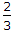# Mechanical Engineering - Hydraulics and Fluid Mechanics

### Exercise :: Hydraulics and Fluid Mechanics - Section 1

21.

The discharge over a rectangular notch is (where b = Width of notch, and H = Height of liquid, above the sill of the notch)

 A.Cd x b2gH B.Cd x b2g x H C.Cd x b2g x H3/2 D.Cd x b2g x H2

Explanation:

No answer description available for this question. Let us discuss.

22.

The discharge through a siphon spillway is

 A. Cd x a2gH B. Cd x a2g x H3/2 C. Cd x a2g x H2 D. Cd x a2g x H5/2

Explanation:

No answer description available for this question. Let us discuss.

23.

The maximum discharge over a broad crested weir is

 A. 0.384 Cd x L x H1/2 B. 0.384 Cd x L x H3/2 C. 1.71 Cd x L x H1/2 D. 1.71 Cd x L x H3/2

Explanation:

No answer description available for this question. Let us discuss.

24.

In a venturimeter, the velocity of liquid at throat is __________ than at inlet.

 A. higher B. lower

Explanation:

No answer description available for this question. Let us discuss.

25.

The loss of head due to friction in a pipe of uniform diameter in which a viscous flow is taking place, is (where RN = Reynold number)

 A. 1/RN B. 4/RN C. 16/RN D. 64/RN